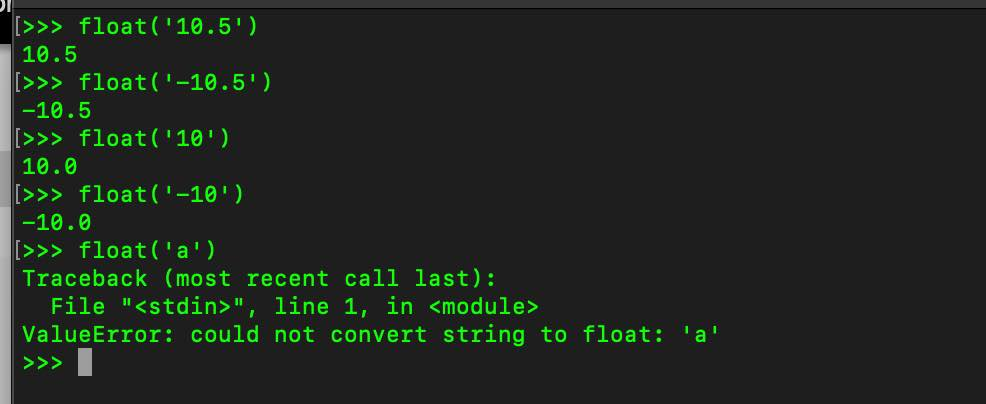# Float built-in function in Python

float() is a part of the built-in function of the Python Interpreter.

Syntax:
``class float(x=0.0)``

The float() function returns a floating point number from the passed in number or string x.

## Example 1: passing a String argument

``````>>> float('10.5')
10.5
>>> float('-10.5')
-10.5
>>> float('10')
10.0
>>> float('-10')
-10.0
>>> float('a')
Traceback (most recent call last):
File "", line 1, in <module>
ValueError: could not convert string to float: 'a'``````

Documentation: https://docs.python.org/3/library/functions.html#float## Example 2: passing exponential values:

``````>>> float('2e3')
2000.0
>>> float('-2e3')
-2000.0``````
Example 3: When no argument is passed

When no argument is passed, the value is set as 0.0

``````>>> float()
0.0``````

## Example 4: NaN and infinity

``````>>> float('NaN')
nan
>>> float('Infinity')
inf
>>> float('-Infinity')
-inf``````

## Python 3 Code example with float()

``````float1 = float(input("Enter float number 1: "))
float2 = float(input("Enter floart number 2: "))

sum_of_two_numbers = float1 + float2

print(f"Sum of {float1} and {float2} is {sum_of_two_numbers}")``````
Output:

Enter float number 1: 1.4
Enter float number 2: 2.4
Sum of 1.4 and 2.4 is 3.8

-

Have Questions? Post them here!